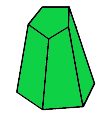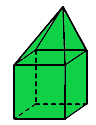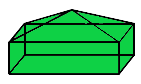Related Articles
Class 8 RD Sharma Solutions – Chapter 19 Visualising Shapes – Exercise 19.1
• Last Updated : 02 Feb, 2021

### Question 1: What is the least number of planes that can enclose a solid? What is the name of the solid?

Solution:

The least number of planes that are required to enclose a solid is 4.

The name of the solid is tetrahedron. It is a solid with four planes.

### (i) 3 triangles?

Solution:

No, because we need minimum 4 triangular faces in order to complete a polyhedron.

### (ii) 4 triangles?

Solution:

Yes, A tetrahedron has 4 triangles as its faces.

### (iii) a square and four triangles?

Solution:

Yes, A square pyramid has a square and four triangles as its faces.

### Question 3: Is it possible to have a polyhedron with any given number of faces?

Solution:

Yes, if the number of faces is equal to or more than 4. As there is no possible polyhedron with 3 or less faces.

### Question 4: Is a square prism same as a cube?

Solution:

Yes, a square prism is same as a cube. Both of them have 6 faces, 8 vertices and 12 edge.
The only difference is that a square prism is a 3-d shape with six rectangular shaped sides, out of which two are squares and
a cube is a rectangular prism having same length, width and height.

### Question 5: Can a polyhedron have 10 faces, 20 edges and 15 vertices?

Solution:

No.

Reason –
Given,
Number of faces (F) = 10
Number of edges (E) = 20
Number of vertices (V) = 15
We know that, every polyhedron satisfies Euler’s formuala.
So, By using Euler’s formula –
V + F = E + 2
15 + 10 = 20 + 2
25 ≠ 22
Since, the given polyhedron does not satisfy Euler’s formula. Thus, a polyhedron can not have 10 faces, 20 edges and 15 vertices.

### Question 6: Verify Euler’s formula for each of the following polyhedrons:

(i)Solution:

In the given polyhedron –
Number of faces (F) = 7
Number of edges (E) = 15
Number of vertices (V) = 10
Now, By using Euler’s formula –
V + F = E + 2
10 + 7 = 15 + 2
17 = 17
Here, Euler’s formula is satisfied.
Hence, verified.

(ii)Solution:

In the given polyhedron –
Number of faces (F) = 9
Number of edges (E) = 16
Number of vertices (V) = 9
Now, By using Euler’s formula –
V + F = E + 2
9 + 9 = 16 + 2
18 = 18
Here, Euler’s formula is satisfied.
Hence, verified.

(iii)(III)

Solution:

In the given polyhedron –
Number of faces (F) = 9
Number of edges (E) = 21
Number of vertices (V) = 14
Now, By using Euler’s formula –
V + F = E + 2
14 + 9 = 21 + 2
23 = 23
Here, Euler’s formula is satisfied.
Hence, verified.

(iv)(IV)

Solution:

In the given polyhedron –
Number of faces (F) = 8
Number of edges (E) = 12
Number of vertices (V) = 6
Now, By using Euler’s formula –
V + F = E + 2
6 + 8 = 12 + 2
14 = 14
Here, Euler’s formula is satisfied.
Hence, verified.

(v)(V)

Solution:

In the given polyhedron –
Number of faces (F) = 9
Number of edges (E) = 16
Number of vertices (V) = 9
Now, By using Euler’s formula –
V + F = E + 2
9 + 9 = 16 + 2
18 = 18
Here, Euler’s formula is satisfied.
Hence, verified.

### Question 7: Using Euler’s formula find the unknown:

Solution:

(i) Given,
Number of vertices (V) = 6
Number of edges (E) = 12
By using Euler’s formula –
V + F = E + 2
6 + F = 12 + 2
F = 14 – 6
F = 8
Thus, the number of faces is 8.

(ii) Given,
Number of faces (F) = 5
Number of edges (E) = 9
By using Euler’s formula –
V + F = E + 2
V + 5 = 9 + 2
V = 11 – 5
V = 6
Thus, the number of vertices is 6.

(iii) Given,
Number of vertices (V) = 12
Number of faces (F) = 20
By using Euler’s formula –
V + F = E + 2
12 + 20 = E + 2
E = 32 – 2
E = 30
Thus, the number of edges is 30.

My Personal Notes arrow_drop_up